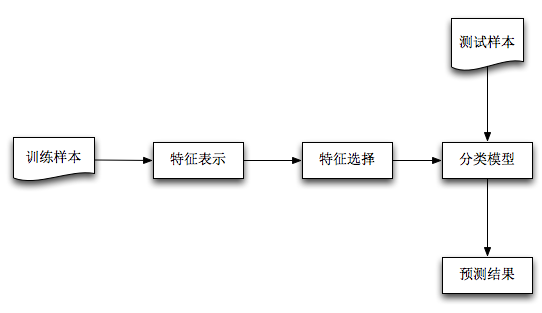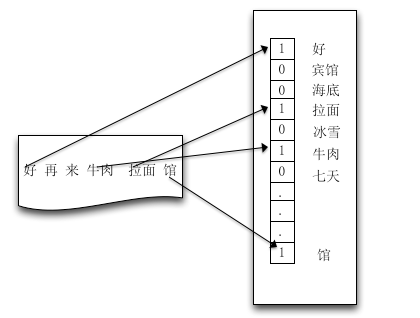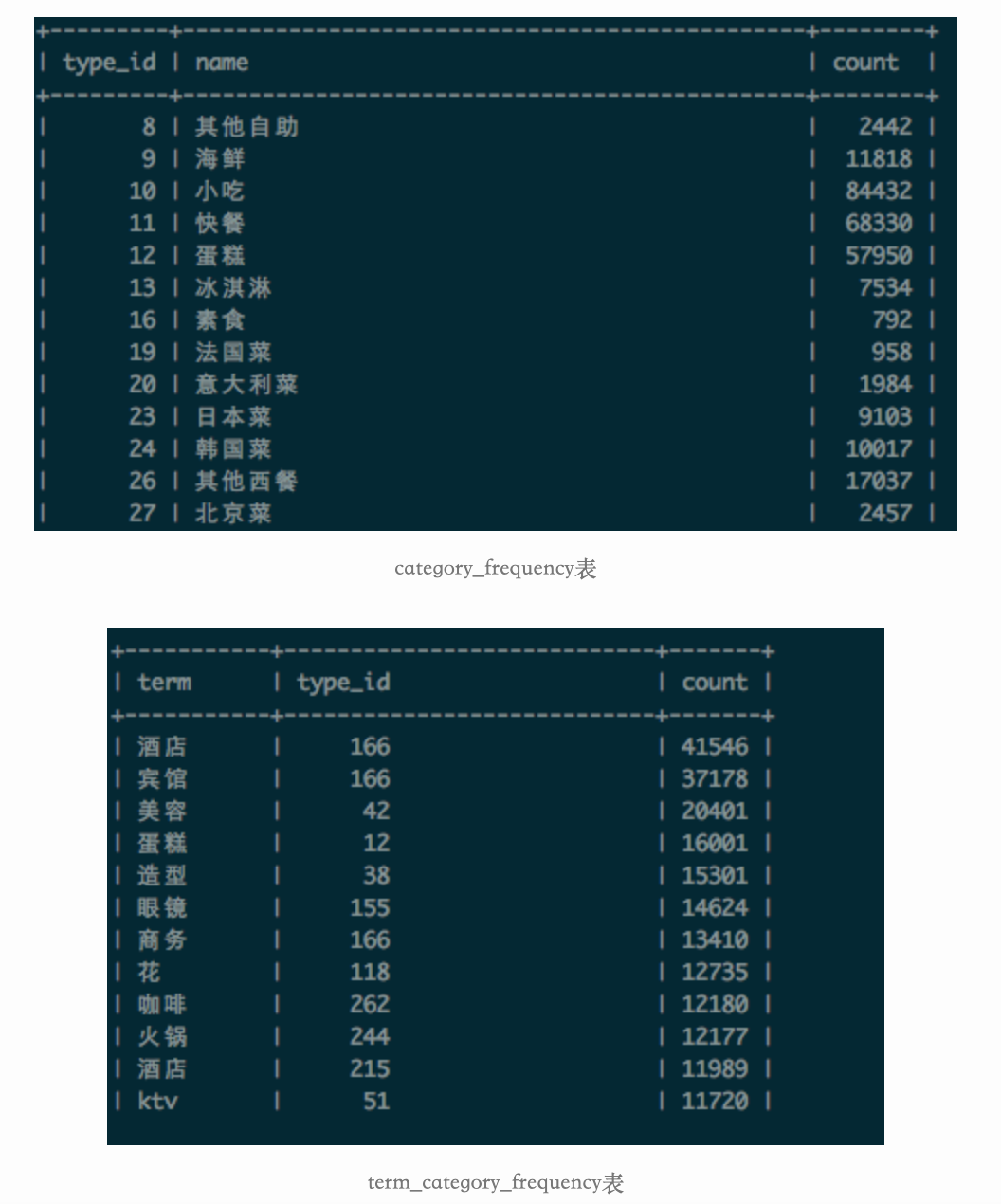## 1. 前言

Note：将商家标示为POI，POI基础信息包括：门店名称、品类、电话、地址、坐标等。## 2. 特征表示Note: 先分词，提取特征词，构造字典，然后使用VSM模型(Vector Space Model)表示文本，生成字典时可以统计词频。

## 3. 特征选择

### 3.1 基于统计学习的特征选择算法

| 米粉(A) | 火锅(B) | 馆(C) | 品类(D) |
| 1 | 1 | 0 | 火锅 |
| 0 | 1 | 1 | 火锅 |
| 1 | 0 | 0 | 小吃 |
| 1 | 0 | 1 | 小吃 |

$$H(Y)=-\sum_{i=1}^{2} p(y_{i}) \log p(y_{i})=-2 * \frac {1} {2} * \log \frac {1} {2} = 1$$

$$H(D|A)=- \sum_{i=1}^{2}\sum_{j=1}^{2}p(x,y)\log p(y|x)=0 - 0 - \frac {1} {2} \log \frac {2} {3} - \frac {1} {4} \log \frac {1} {3} =0.69$$

### 3.2 基于领域知识的特征选择方法

Note: 利用领域知识集合统计学习来选择特征。